# Min and max

Lesson contents

Often you want to find the smallest (minimum) and largest (maximum) values. For example, the goat with the smallest weight, and the goat with the largest weight. This lesson is about how you do that.

We'll use the same data as before.

• "Dob",12,"Yes"
• "Mug",21,"Yes"
• "Mop",44,"No"
• "Jib",18,"Yes"

The first field is goat name. The second is goat weight. The last is whether the goat like Borderlands.

You can download the sample min/max workbook, and the CSV data file.

Let's start by finding the heaviest goat, when the data is in arrays.

### Data in arrays

#### Heaviest goat

As before, we're going to loop across the arrays.

• For sCurrentRecord = 1 To sNumberRecords
•     Do stuff with atGoatNames(sCurrentRecord), asGoatWeights(sCurrentRecord), and atGoatLikesBorderlands(sCurrentRecord)
• Next sCurrentRecord

Let's do this:

• Dim sMaximumWeight as Single
• ...
•
• For sCurrentRecord = 1 To sNumberRecords
•     If asGoatWeights(sCurrentRecord) > sMaximumWeight Then
•         sMaximumWeight = asGoatWeights(sCurrentRecord)
•     End If
• Next sCurrentRecord
•
• MsgBox "Maximum weight: " & sMaximumWeight

Each time through the loop, we compare the weight of the current goat with the `sMaximumWeight` we've found so far. If the weight of the current goat is more than `sMaximumWeight`, we replace `sMaximumWeight` with the weight of the current goat.

Let's try it.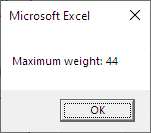Yay! It worked.

(Actually, it has a subtle problem. More later.)

#### Lightest goat

Let's try this:

• Dim sMinimumWeight as Single
• ...
•
• For sCurrentRecord = 1 To sNumberRecords
•     If asGoatWeights(sCurrentRecord) < sMinimumWeight Then
•         sMinimumWeight = asGoatWeights(sCurrentRecord)
•     End If
• Next sCurrentRecord
•
• MsgBox "Minimum weight: " & sMinimumWeight

Each time through the loop, we compare the weight of the current goat with the `sMinimumWeight` we've found so far. If the weight of the current goat is less than `sMinimumWeight`, we replace `sMinimumWeight` with the weight of the current goat.

OK, let's try it. We get: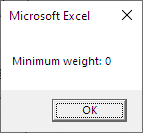Wait, what!? The lightest goat is weightless?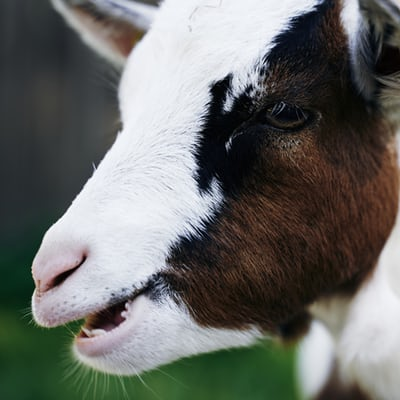That doesn't look right.

Let's check the data:

• "Dob",12,"Yes"
• "Mug",21,"Yes"
• "Mop",44,"No"
• "Jib",18,"Yes"

That isn't right. The lightest is 12.

Hey, Georgina. Can you work out the problem?Georgina

Sure. I'll add a breakpoint in the processing loop. Let's see what happens.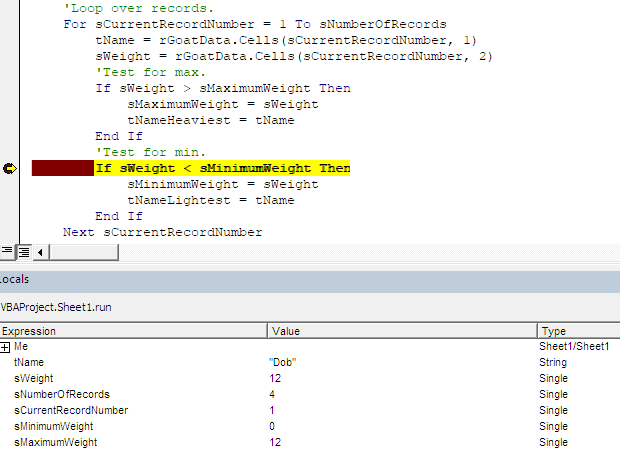The first time through the loop, `sCurrentRecord` is 1, and `asGoatWeights(currentRecord)` is 12.Georgina

OK, I see the problem. The `If` is comparing the goat weight (that's 12) with zero.

• If asGoatWeights(sCurrentRecord) 12 < sMinimumWeight 0 Then

All of the weights are more than zero, so `sMinimumWeight` is never changed.Ray

Why is `sMinimumWeight` zero?

Wait..., never mind. It starts out at zero. That's what all variables start out as. Well, all `Single`s, anyway.

Right. The problem is that `sMinimumWeight` starts out at 0, which is smaller than the actual smallest value in the data, so it never gets changed by the `If` statement.

To fix it, let's initialize `sMinimumWeight` to a very large value. All of the weights in the arrays will be less than that.

• Dim sMinimumWeight as Single
• ...
• sMinimumWeight = 3.4028235E+38
• For sCurrentRecord = 1 To sNumberRecords
•     If asGoatWeights(sCurrentRecord) < sMinimumWeight Then
•         minimumWeight = asGoatWeights(sCurrentRecord)
•     End If
• Next sCurrentRecord
•
• MsgBox "Minimum weight: " & sMinimumWeight

`3.4028235E+38` is the largest value a `Single` can hold. It's 34,028,235,000,000,000,000,000,000,000,000,000,000. The `E` means "times ten to the".

Let's see if that works.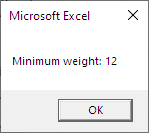Hooray!Adela

Should we make the same change to the maximum code? Just in case.

Oh, right! Yes, we should. For maximum weights, it doesn't make much difference. `sMaximumWeight` starts off at zero, and all of the goat weights will be more than that.

But if we were dealing with, say, temperature data for different places, it's possible that all of the temperatures would be negative. Then we'd get zero for the maximum, rather than the right value.

So let's initialize `sMaximumWeight` to the smallest possible value. Every value will be bigger than that.

• Dim sMaximumWeight as Single
• ...
• sMaximumWeight = -3.4028235E+38
• For sCurrentRecord = 1 To sNumberRecords
•     If asGoatWeights(sCurrentRecord) > sMaximumWeight Then
•         sMaximumWeight = asGoatWeights(sCurrentRecord)
•     End If
• Next sCurrentRecord
•
• MsgBox "Maximum weight: " & sMaximumWeight

#### Which goat?

What if we want to know the name of the heaviest goat? No worries.

• Dim sMaximumWeight as Single
• Dim tHeaviestGoat as String
• ...
• sMaximumWeight = -3.4028235E+38
• tHeaviestGoat = ""
• For sCurrentRecord = 1 To sNumberRecords
•     If asGoatWeights(sCurrentRecord) > sMaximumWeight Then
•         sMaximumWeight = asGoatWeights(sCurrentRecord)
•         tHeaviestGoat = atGoatNames(sCurrentRecord)
•     End If
• Next sCurrentRecord
•
• MsgBox tHeaviestGoat & " is the heaviest goat, weighing " & sMaximumWeight & " kilos."

We could do the same thing for the lightest.Marcus

OK, what if you want the lightest goat, and the heaviest goat. Would you have two separate loops, or put everything into one loop?

I'd put everything into one loop. Like this, that also computes the total and average weight.

• sMinimumWeight = 3.4028235E+38
• tLightestGoat = ""
• sMaximumWeight = -3.4028235E+38
• tHeaviestGoat = ""
• sTotalWeight = 0
• 'Loop over records.
• For sCurrentRecord = 1 To sNumberRecords
•     'Add to total.
•     sTotalWeight = sTotalWeight + asGoatWeights(sCurrentRecord)
•     'Test for min and max.
•     If asGoatWeights(sCurrentRecord) < sMinimumWeight Then
•         sMinimumWeight = asGoatWeights(sCurrentRecord)
•         tLightestGoat = atGoatNames(sCurrentRecord)
•     End If
•     If asGoatWeights(sCurrentRecord) > sMaximumWeight Then
•         sMaximumWeight = asGoatWeights(sCurrentRecord)
•         tHeaviestGoat = atGoatNames(sCurrentRecord)
•     End If
• Next sCurrentRecord
• sAverageWeight = sTotalWeight / sNumberRecords

### Data in ranges

We've looked at getting the largest and smallest for data in arrays. But what if the data is in a `Range`?

It's the same thing. Except that instead of getting data from an array, it comes from a range. So:

• 'Initialize.
• sMinimumWeight = 3.4028235E+38
• sMaximumWeight = -3.4028235E+38
• ...
• For sCurrentRecord = 1 to sNumberRecords
•     'Get the data from a Range, rather than an array.
•     'This is the only line that's different.
•     sWeight = rGoatDataTable.Cells(sCurrentRecord, FIELD_WEIGHT)
•     If sWeight < sMinimumWeight Then
•         sMinimumWeight = sWeight
•     End If
•     If sWeight > sMaximumWeight Then
•         sMaximumWeight = sWeight
•     End If
• Next sCurrentRecord

Now we have a pattern for min/max.

Pattern

Loop across some records. Put an `If` in the loop, comparing the current value with the smallest/largest so far.

### Exercise

Exercise

Dancing with the goats

Download the starting workbook. It has data on scores from an episode of the new hit TV show, "Dancing with the Goats."

It starts like this: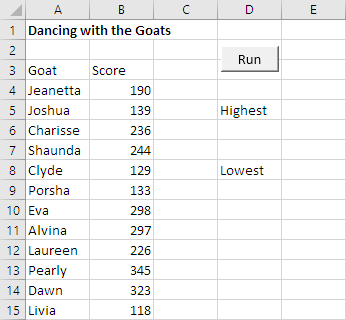Click the button, and see the goats with the highest and lowest scores.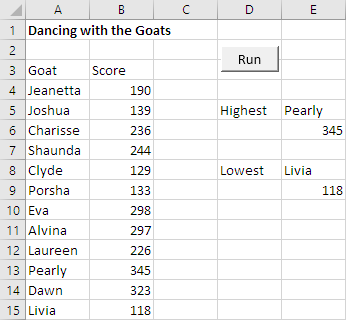Write the code so it would still work if the number of records changed.

Upload your workbook. The usual standard apply.

### Up next

Let's watch Marcus write some code.

Attachments